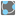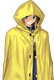• # Formatting... welp (help!)

## Question related to missionThree Points Circle

I would like to do this elegantly, where my last return looks like that:

```return "(x{:+.2f})^2+(y{:+.2f})^2={:.2f}^2".format(-round(middle, 2), -round(middle, 2), round(distance((points, middle)), 2))
```

This would be allright if not the fact, that {.2f} is fixed formatting of 2 digits behind the point. What should I use to get not exactly, but only if needed 2 digits behind the point? It's hard for me to google it out.

``` Your result: "(x-3.00)^2+(y-3.00)^2=1.41^2"
Right result: "(x-3)^2+(y-3)^2=1.41^2"
```

:C12